## LetsPlayMaths.Com

WELCOME TO THE WORLD OF MATHEMATICS

# Class 4 Decimal Number

Introduction to Decimals

Tenths

Decimals and Whole Numbers

Hundredths

Thousandths

Exapanded form of Decimal Numbers

Equivalent Decimal Numbers

Like and Unlike Decimal Numbers

Conversion of Unlike Decimal Numbers into Like Decimal Numbers

Subtraction of Decimal Numbers

Multiplication of Decimal Numbers by 10,100,1000

Division of Decimal Numbers by 10,100,1000

Money and Decimals

Subtraction of Money

Multiplication of Money

Division of Money

Decimals Test

Decimals Worksheet

## Introduction to Decimals

We know that the place value of digit increases ten times as it moves one step towards left. Let's have a look at some examples.

Number Place Value of 5 Increment
6725 5 5 X 1
6752 50 5 X 10
6572 500 5 X 10 X 10
5672 5000 5 X 10 X 10 X 10

What will happen to the place value of a digit when it moves one step towards right of it's unit place. The place value of a digit becomes 110 (One Tenth), let's have a look at some examples.

Position of a number Place Value of 5 Decrement
5 in unit place 5 5 X 1
5 moves one place to right 0.5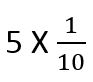5 moves two places to right 0.005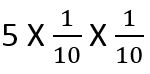5 moves three places to right 0.0005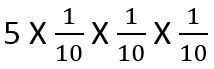## Tenths

Have a look at the below mentioned figure.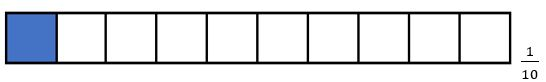Above figure is divided into ten equal parts, out of these one part is shaded. This shaded part represents one tenth of the whole figure. It is written as 110 or 0.1.

The following figure is divided into ten equal parts, and 3 parts are shaded. These shaded parts represent 310 or 0.3.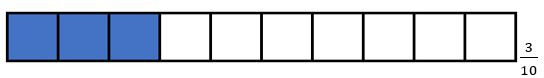## Decimals and Whole Numbers

Whole numbers can be written with decimal point. Few examples are given below.

1 = 1.0     2 = 2.     5 = 5.0     12 = 12.0

Proper fraction can have only decimal points, let’s see some examples.Mixed fractions must have a whole number as well as decimal with it. Let’s have a look at some examples.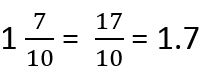Here, 1 is the whole number and 0.7 is the decimal number.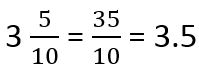Here, 3 is the whole number and 0.5 is the decimal number.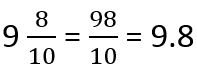Here, 9 is the whole number and 0.8 is the decimal number.

## HundredthsAbove figure is divided into one hundred equal parts, and one part is shaded. The Shaded part represents
one hundredth (1100) of the whole figure.
1100 can be written as 0.01 and it is read as Zero Decimal Zero one or Zero point Zero one.

Let's have a look at similar examples.

8100 = 0.08 (Zero point zero eight / Zero decimal zero eight)

15100 = 0.15 (Zero point one five / Zero decimal one five)

87100 = 0.87 (Zero point eight seven / Zero decimal eight seven)

265100 = 265100 = 2.65 (Two point six five / Two decimal six five)

## Thousandths

If we divide a square into one thousand equal parts, then one small part will be known as one thousandth or 11000 or 0.001.

It is read as Zero point zero zero one or Zero Decimal zero zero one.

81000 = 0.008 (Zero point zero zero eight / Zero decimal zero zero eight)

151000 = 0.015 (Zero point zero one five / Zero decimal zero one five)

871000 = 0.087 (Zero point zero eight seven / Zero decimal zero eight seven)

2651000 = 0.265 (Zero point two six five / Zero decimal Two six five)

The digits after the decimal point are read one by one. For example, 0.578 is read as zero point five seven eight.

## Exapanded form of Decimal Numbers

Let's see some examples of expanded form of decimal numbers.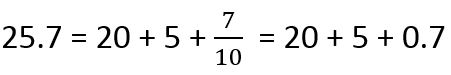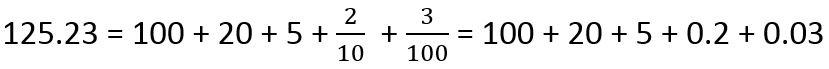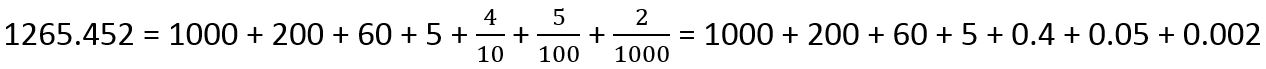## Equivalent Decimal Numbers

In decimal number comparison, we first compare the whole number first. If the whole number parts are equal, then we compare the extreme left digits of the decimal parts. If they are equal, then we compare the next digits and so on. Let's see some examples.

Example 1. Which decimal number is greater between 62.45 and 75.05?

Solution. Whole number of 62.45 = 62

Whole number of 75.05 = 75

We know 75 is greater than 62.

So, 75.05 > 62.45

Example 2. Which decimal number is greater between 100.65 and 100.28?

Solution. Here both decimal numbers are having same whole number, that is 100. Now, we must consider

1st decimal digits for comparison.

0.6 > 0.2

So, 100.65 > 100.28

Let's consider a fraction having 10 in the denominator, that is 510.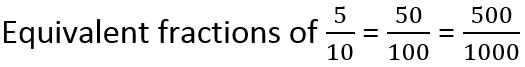Now convert the above fractions into decimal format.

0.5 = 0.50 = 0.500

So, 0.5 equivalent decimals are 0.50, 0.500, 0.5000 ... so on.

From above we came to know that by adding any numbers of zeros to the extreme right digit of the decimal number does not change the value of decimal number.

Example 1. What are the equivalent decimals of 0.8?

Solution. We must add zeros to the extreme right of the decimal digit.

0.8 = 0.80 = 0.800 = 0.8000

Equivalent decimals of 0.8 = 0.80, 0.800, 0.8000... so on.

Example 2. What are the equivalent decimals of 1.25?

Solution. Equivalent decimals of 1.25 = 1.250, 1.2500, 1.25000... so on.

## Like and Unlike Decimal Numbers

Like and unlike decimal numbers are similar to Like and unlike fractions. Let's have a look at some examples.

510, 710, 810, 910, 1510 are like fractions.

If we convert these like fractions to decimals, then they will be known as like decimal numbers.

0.5, 0.7, 0.8, 0.9, 1.5 are like decimal numbers.

510, 7100, 1251000 are unlike fractions.

If we convert these unlike fractions into decimal format, then they will become unlike decimals.

0.5, 0.07, 0.125 are unlike decimal numbers.

## Conversion of Unlike Decimal Numbers into Like Decimal Numbers

While converting unlike decimals into like decimals we must do following things. Let us consider unlike decimal 0.8 and 0.315 for conversion.

1. count the digits present after the decimal point for both the numbers and find out the difference.

Number of decimal digits present in 0.8 = 1

Number of decimal digits present in 0.315 = 3

Difference in decimal digits between two decimal numbers = 3 − 1 = 2

2. Add that much zeros (Difference of decimal digits) to the extreme right of the decimal number which is having less decimal digits.

Here 0.8 is having less decimal digits.

Add two zeros to the extreme right of 0.8 = 0.800

Now, 0.800 and 0.315 became like decimal numbers.

Decimal number addition is like our normal addition. But, here we must follow certain rules that are mentioned below.

1. First convert all the decimals to like decimals.

2. Write the decimal numbers in column format. Unit place should come in one column, tens place should come in one column, hundred place should come in one column, thousand place should come in one column and so on. Decimal point should come in one column, tenth come in one column, hundredth come in one column and so on.

3. Do the normal addition keeping decimal point between whole and decimal number.

Let's see some examples.

Example 1. Add 0.25 and 0.1.

Solution.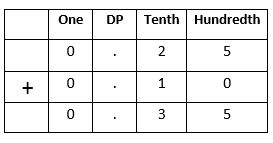Example 2. Add 25.37 and 10.05

Solution.Example 3. Add 54.1 and 6.95

Solution.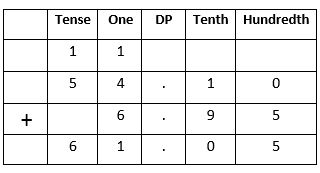## Subtraction of Decimal Numbers

Decimal number subtraction is like our normal subtraction. But, here we must follow certain rules that are mentioned below.

1. First convert all the decimals to like decimals.

2. Write the decimal numbers in column format. Unit place should come in one column, tens place should come in one column, hundred place should come in one column, thousand place should come in one column and so on. Decimal point should come in one column, tenth come in one column, hundredth come in one column and so on.

3. Do the normal subtraction keeping decimal point between whole and decimal number.

Let's see some examples.

Example 1. Subtract 5.4 from 8.5.

Solution.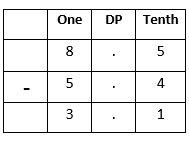Example 2. Subtract 10.74 from 125.51.

Solution.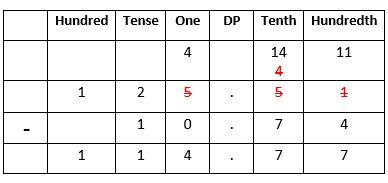## Multiplication of Decimal Numbers by 10,100,1000

If we multiply a decimal number by 10, then the decimal point will be shifted one place to the right.

Example 1. Multiply 10 by 0.572.

Solution.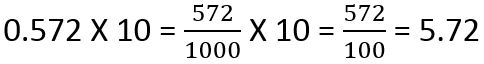Example 2. Multiply 10 by 67.54.

Solution.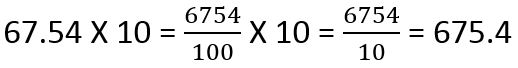If we multiply a decimal number by 100, then the decimal point will be shifted two places to the right.

Example 1. Multiply 100 by 0.892.

Solution.Example 2. Multiply 100 by 78.325.

Solution.If we multiply a decimal number by 1000, then the decimal point will be shifted three places to the right.

Example 1. Multiply 1000 by 0.2365.

Solution.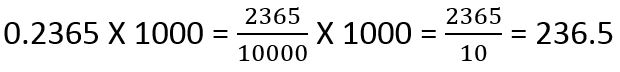Example 2. Multiply 1000 by 23.64.

Solution.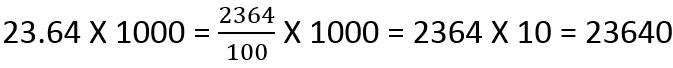## Division of Decimal Numbers by 10, 100, 1000

If we divide a decimal number by 10, then the decimal point will be shifted one place to the left.

Example 1. Divide 5.76 by 10.

Solution.Example 2. Divide 462 by 10.

Solution.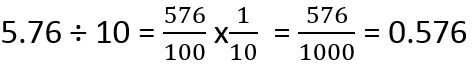If we divide a decimal number by 100, then the decimal point will be shifted two places to the left.

Example 1. Divide 127.37 by 100.

Solution.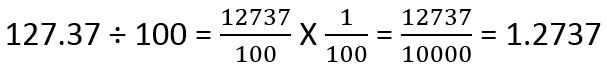Example 2. Divide 92.85 by 100

Solution.If we divide a decimal number by 1000, then the decimal point will be shifted three places to the left.

Example 1. Divide 9.05 by 1000.

Solution.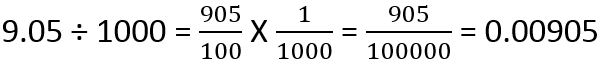Example 2. Divide 52 by 1000.

Solution.## Money and Decimals

100 Paisa make one rupee.

That means, 100 Paisa = 1 Rupee

1 Paisa = 1 hundredth of a rupee = 1/100 Rupee = 0.01 Rupee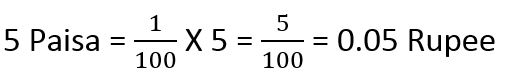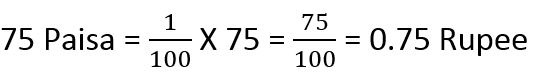We use two digits to represent paisa while writing in decimal form. For example, 10 rupees 5 paisa is written as Rs. 10.05 and 75 rupees 65 paisa is written as Rs. 75.65.

Addition of money is like addition of decimals and whole numbers. Let's have a look at some examples.

Example 1. Add 5 rupees and 65 paisa to 6 rupees.

Solution. Write both the figures in tabular format as shown below.So, the answer is 11 rupees 65 paisa.

Example 2. Add Rs. 34.25 and 36.75.

Solution. Write both the figures in tabular format as shown below.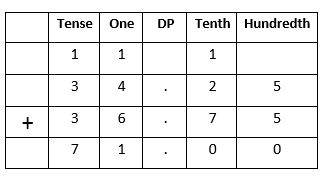So, the answer is 71 rupees.

Example 3. Add Rs. 145.21, Rs. 321 and Rs. 45.67.

Solution.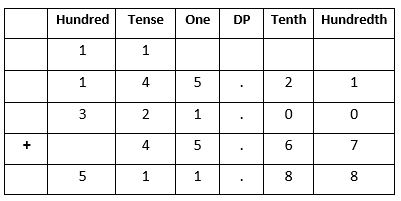So, the answer is 511 rupees 88 paisa.

## Subtraction of Money

Subtraction of money is like subtraction of decimals and whole numbers. Let's have a look at some examples.

Example 1. Subtract Rs. 34.75 from Rs. 78.25.

Solution. Write both the figures in tabular format as shown below.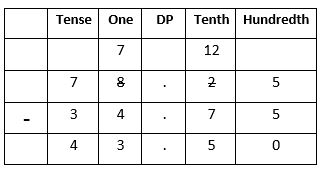So, the answer is Rs. 43.50

Example 2. John has Rs 500 with him. He bought few books for Rs. 278.25 and purchased movie ticket for Rs. 75. How much money remained with him?

Solution. Total money spent by John = Rs. 278.25 + Rs. 75Money remained with John = Rs. 500 – Rs. 353.25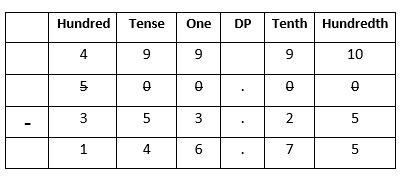So, John had Rs. 146.75 with him.

## Multiplication of Money

Multiplication of money will be like normal multiplication, but here we must put a decimal point two place from extreme right. Let's have a look at some examples.

Example 1. A cricket bat costs Rs. 127.25. What is the cost of 5 bats?

Solution. One cricket bat costs Rs. 127.25.

5 bats will cost = Rs. 127.25 X 5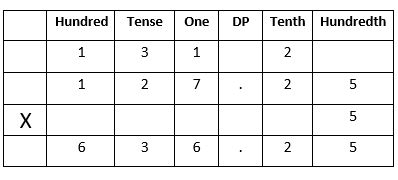So, 5 cricket bats will cost Rs. 636.25.

Example 2. Ram bought 4 kg of rice and 8 kg of sugar from a shop. Cost of rice is Rs. 52.45 per kg and sugar is Rs.24.25 per kg. How much money Ram must pay the shopkeeper?

Solution. 1 kg rice cost Rs. 52.45.

Cost of 4 kg rice = Rs. 52.45 × 4 = Rs. 209.80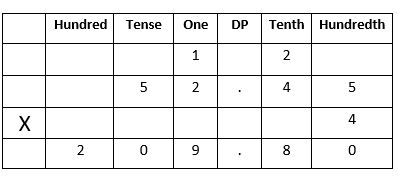1 kg sugar cost Rs. 24.25. Cost of 8 kg sugar = Rs. 24.25 × 8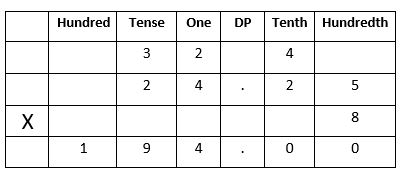Total cost of 4 kg rice and 8 kg sugar = Rs. 209.80 + Rs. 194 = Rs. 403.80

So, Ram must pay Rs. 403.80 to the shopkeeper.

## Division of Money

Division of money is like normal division, but we must put decimal point two place from extreme right. Let's have a look at some examples.

Example 1. Bob bought 6 notebooks for Rs. 321.24. what is the cost of each notebook?

Solution. 6 notebooks cost = Rs. 321.24

1 notebook will cost = Rs. 321.24 ÷ 6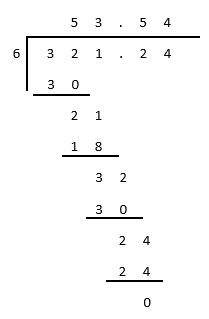So, cost of one notebook is Rs. 53.54.

Example 2. Divide Rs. 26.15 by 5.

Solution.So, the answer is Rs. 5.23.

## Decimals Test

Decimals Test - 1

Decimals Test - 2

## Class-4 Decimals Worksheet

Decimals Worksheet - 1

Decimals Worksheet - 2

Decimals Worksheet - 3

Decimals Worksheet - 4

Money Decimals Worksheet - 1

Money Decimals Worksheet - 2

Money Decimals Worksheet - 3